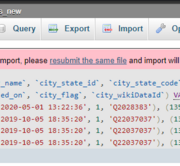# How Can I Convert a String in PHP Into an Array?

We will go over how to transform a string into an array in PHP in this little tutorial. Describe how to convert a string value into an array in PHP step by step. I’d like to share with you how to transform a string using a comma in PHP. Learn how to use a delimiter in PHP to transform a string into an array. To learn how to generate an array in PHP from a string, follow the steps below.

We’ll utilize PHP’s explode() function to transform a string into an array. Using a delimiter, explode() offers methods for turning a string into an array. In order for you to comprehend how it functions, let me give you two instances.

## Example:- 1

``````<?php

\$myString = "Hi, I am devopsschool.com";

\$array = explode(' ', \$myString);

print_r(\$array);

?>``````

## Example:- 2

``````<?php

\$myString = "Rajkot,Surat,Bhavnagar,Baroda";

\$array = explode(',', \$myString);

print_r(\$array);

?>``````

## PHP explode() Function Example Tutorial

A PHP explode function example is given in this article. I will demonstrate how to use PHP’s explode string to array function. This is a basic example…

## How to Convert Array to String in PHP?

We will cover the example of converting an array to a string in PHP in this lesson. We’ll examine an example of converting an array to a…

## How to Use the array_merge() Function in PHP?

We will go over the simple and quick method to use the PHP array_merge() function example in this brief tutorial. Let’s talk about the PHP example of…

## How to Merge Two Arrays in PHP?

I explained simply how to merge two arrays in PHP Laravel. you will learn how to append two arrays in PHP. you will learn how to merge…

## How to Get the First 5 Elements of Array in PHP?

Here, I will show you the php array to get the first 5 elements. let’s discuss about php array getting 5 first element values. you can see…In this article we have shown How to fix the “Script timeout passed, if you want to finish import, please resubmit the same file and import will…